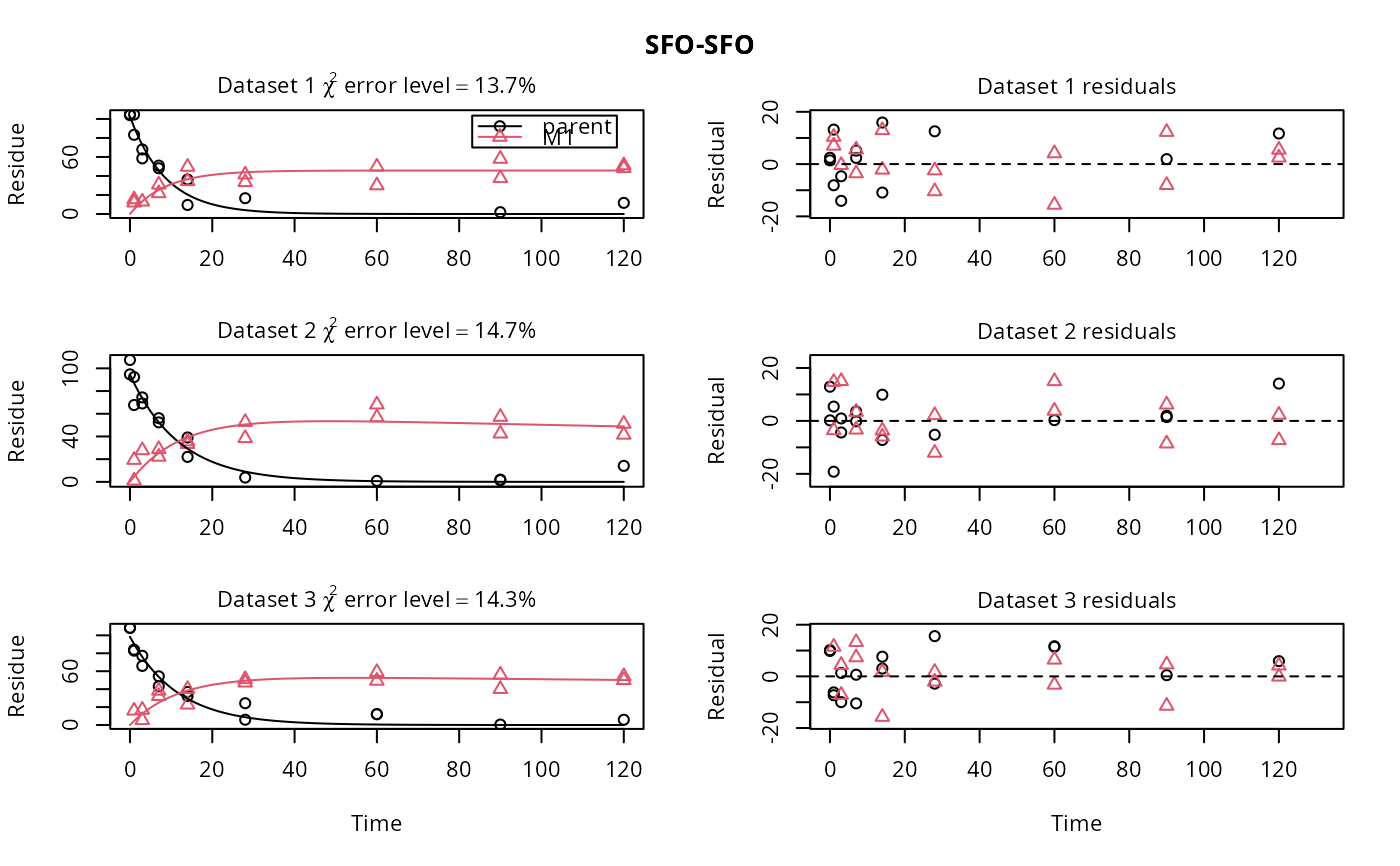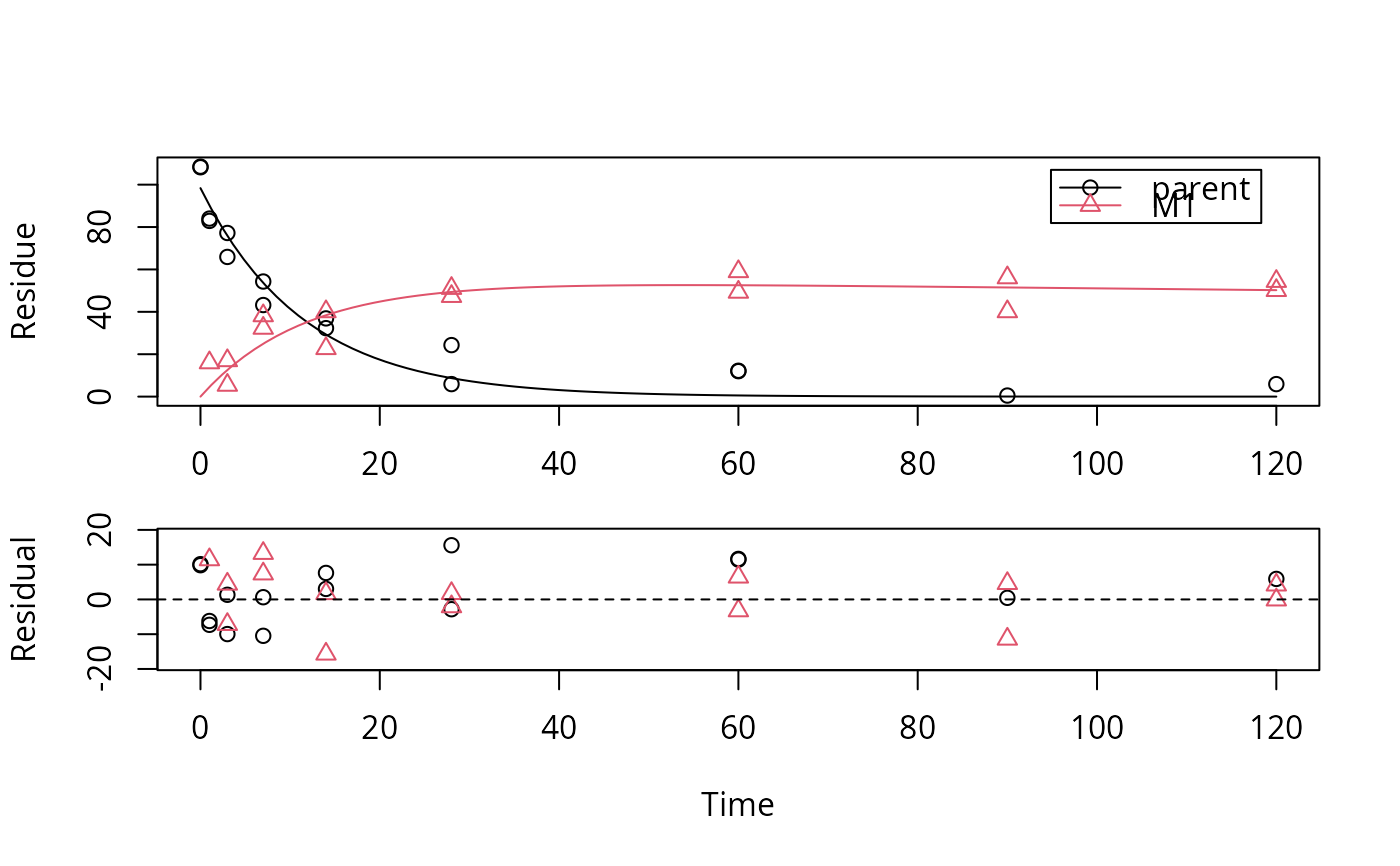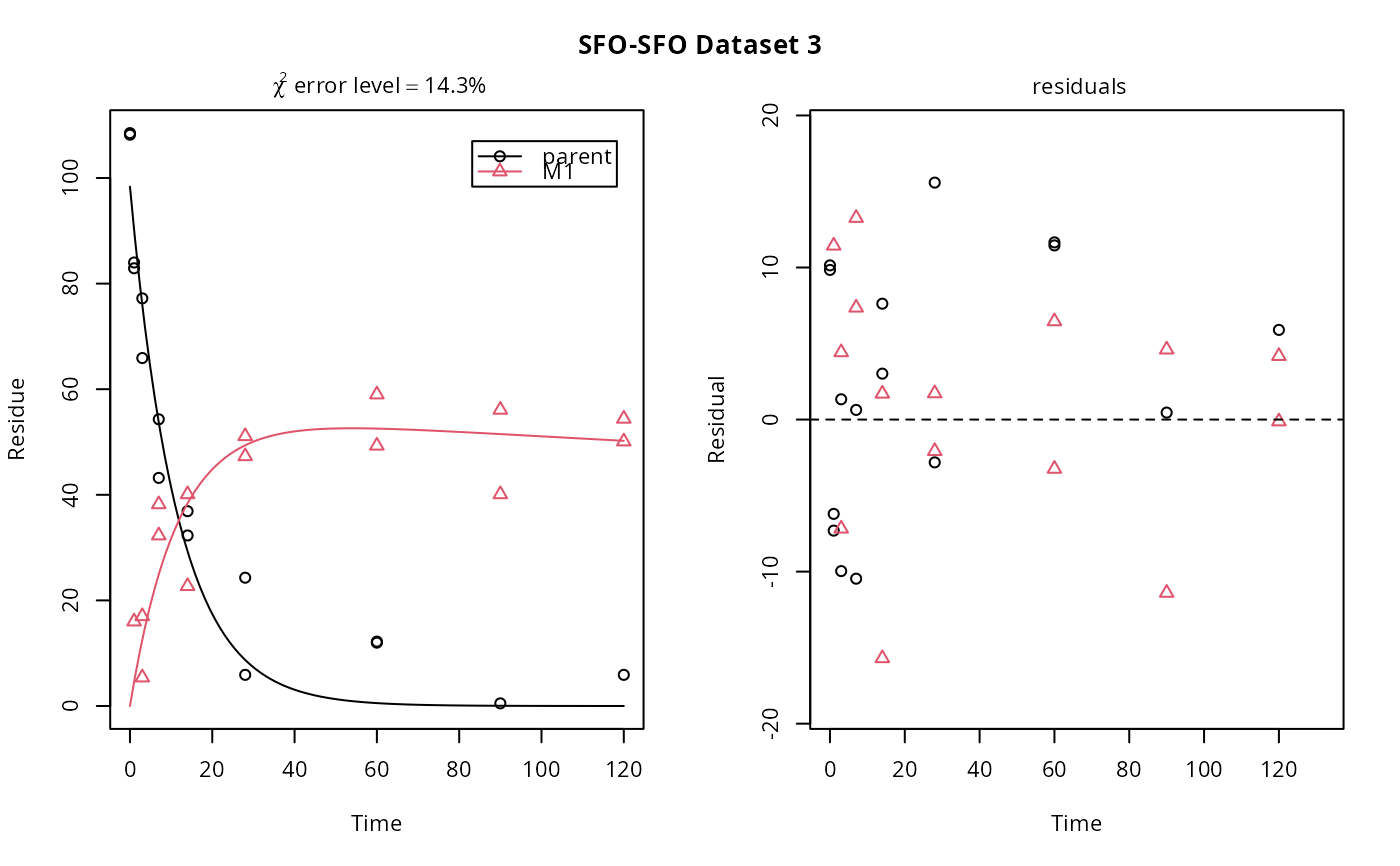Normally distributed errors are added to data predicted for a specific degradation model using mkinpredict. The variance of the error may depend on the predicted value and is specified as a standard deviation.

add_err(
prediction,
sdfunc,
secondary = c("M1", "M2"),
n = 10,
LOD = 0.1,
reps = 2,
digits = 1,
seed = NA
)

## Arguments

prediction

A prediction from a kinetic model as produced by mkinpredict.

sdfunc

A function taking the predicted value as its only argument and returning a standard deviation that should be used for generating the random error terms for this value.

secondary

The names of state variables that should have an initial value of zero

n

The number of datasets to be generated.

LOD

The limit of detection (LOD). Values that are below the LOD after adding the random error will be set to NA.

reps

The number of replicates to be generated within the datasets.

digits

The number of digits to which the values will be rounded.

seed

The seed used for the generation of random numbers. If NA, the seed is not set.

## Value

A list of datasets compatible with mmkin, i.e. the components of the list are datasets compatible with mkinfit.

Johannes Ranke

## Examples


# The kinetic model
m_SFO_SFO <- mkinmod(parent = mkinsub("SFO", "M1"),
M1 = mkinsub("SFO"), use_of_ff = "max")
#> Temporary DLL for differentials generated and loaded

# Generate a prediction for a specific set of parameters
sampling_times = c(0, 1, 3, 7, 14, 28, 60, 90, 120)

# This is the prediction used for the "Type 2 datasets" on the Piacenza poster
# from 2015
d_SFO_SFO <- mkinpredict(m_SFO_SFO,
c(k_parent = 0.1, f_parent_to_M1 = 0.5,
k_M1 = log(2)/1000),
c(parent = 100, M1 = 0),
sampling_times)

# Add an error term with a constant (independent of the value) standard deviation
# of 10, and generate three datasets
d_SFO_SFO_err <- add_err(d_SFO_SFO, function(x) 10, n = 3, seed = 123456789 )

# Name the datasets for nicer plotting
names(d_SFO_SFO_err) <- paste("Dataset", 1:3)

# Name the model in the list of models (with only one member in this case) for
# nicer plotting later on.  Be quiet and use only one core not to offend CRAN
# checks
# \dontrun{
f_SFO_SFO <- mmkin(list("SFO-SFO" = m_SFO_SFO),
d_SFO_SFO_err, cores = 1,
quiet = TRUE)

plot(f_SFO_SFO)# We would like to inspect the fit for dataset 3 more closely
# Using double brackets makes the returned object an mkinfit object
# instead of a list of mkinfit objects, so plot.mkinfit is used
plot(f_SFO_SFO[], show_residuals = TRUE)# If we use single brackets, we should give two indices (model and dataset),
# and plot.mmkin is used
plot(f_SFO_SFO[1, 3])# }